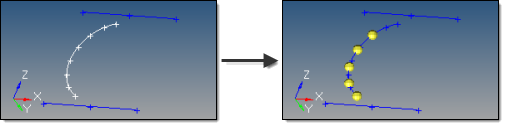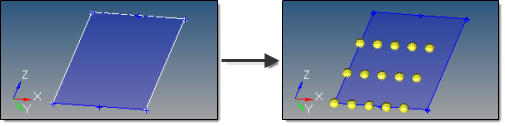﻿ extract parametric subpanel (Nodes panel)
Manufacturing Solutions

# extract parametric subpanel (Nodes panel)# extract parametric subpanel (Nodes panel)This subpanel creates nodes at parametric locations on lines and surfaces.

### For lines:

 • 0.0 <= u lower bound <= u upper bound <= 1.0.
 • If the lower and upper bounds are the same, only one node is created.
 • If the number of u nodes is specified as 1, only the lower bound is used.
 • The line parameterization type can be specified as either arc length or internal.  Internal parameterizations depend on how the line was originally created, while arc length simply distributes the nodes uniformly.This example uses u lower bound 0, u upper bound 0.75, u nodes = 5, and arc length parameterization.

### For surfaces:

 • 0.0 <= u lower bound <= u upper bound <= 1.0.
 • 0.0 <= v lower bound <= v upper bound <= 1.0.
 • The number of u and v nodes must be >= 1.
 • The total number of nodes created equals (number of u nodes * number of v nodes).
 • The surface parametric area is scaled to the visible surface area. Nodes will be created inside the visible surface area.Here, u ranges from 0 to 0.75 with 5 nodes, while v ranges from 0 to 0.66 with 3 nodes.